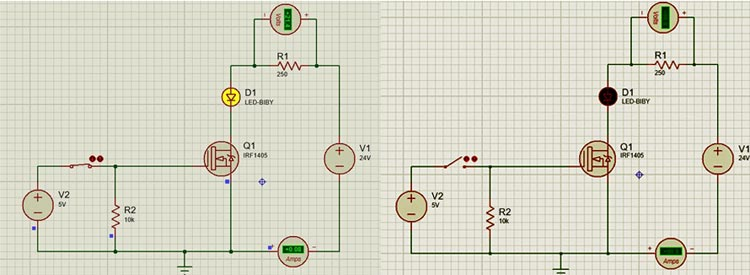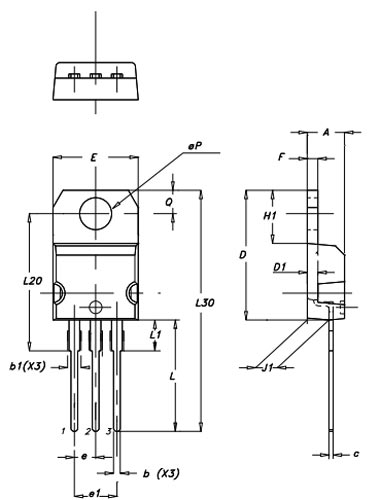# P55NF06 N-Channel Power MOSFET

The P55NF06 is an N-channel MOSFET with a high drain current of 50A and a low Rds value of 18 mΩ. It also has a VGS of 20V at which the MOSFET will start conducting. Hence it is commonly used to drive applications. However, a driver circuit is needed if the MOSFET has to be switched in completely.

### P55NF06 MOSFET Pinout Configuration

 Pin Number Pin Name Description 1 Gate Controls the biasing of the MOSFET 2 Drain Current flows in through the Drain 3 Source Current flows out through Source at leaves MOSFET

### Features & Specifications

• N-Channel Power MOSFET
• Continuous Drain Current (ID): 35A
• Pulsed Drain Current (ID-peak) is 50A
• Drain to Source Breakdown Voltage: 60V
• Drain Source Resistance (RDS) is 0.018 Ohms
• Gate threshold voltage (VGS-th) is 20V (max)
• Rise time and fall time is 50nS and 15nS
• Input Capacitance 1300pF
• Output Capacitance 300pF
• Available in To-220 package

Note: Complete technical details can be found in the P55NF06 datasheet given at the bottom of the page

### P55NF06 Equivalent MOSFETs

IRF2807, IRFB3207, IRFB4710, IRFZ44N, IRF1405, IRF540N, IRF3205

### General Description of P55NF06 MOSFET

The P55NF06 MOSFET is an N-channel power MOSFET that can be used in many different applications. This MOSFET is known for its high drain current and fast switching speed on top of that it has a very low on-resistance that increases the efficiency of this device. According to the datasheet, this MOSFET will start conducting with a small gate voltage of 10V, but the max drain current will flow when the gate voltage is 20V. As this MOSFET is designed for high-power applications this can be driven by a microcontroller or a logic level device.

This MOSFET has a low gate threshold voltage of only 4V, this means that the MOSFET can be turned on even with 5V from the GPIO pin of microcontrollers like Arduino. But this does not mean that the MOSFET will turn on completely with just 5V, it needs around 10V supplied to the gate pin to turn on completely and supply 27A constant current. So, if you are looking for a MOSFET to be used with a microcontroller then you should consider the logic-level MOSFET like 2N7002 or IRLZ44N etc. Alternatively, you can also use a driver circuit to provide 10V to the gate pin of this MOSFET using a transistor. The frequency response of this MOSFET is quite good hence it can be used in DC-DC converter circuits also.

Under normal circumstances and without external influence there will no voltage present in the gate of the MOSFET because the MOSFET is consist of a capacitor and that is why we need to pull down the gate of the MOSFET to the ground in order to prevent false triggering of the circuit, as we all know that MOSFET are voltage-controlled devices which means to turn off the MOSFET we need to provide only voltage to the gate and theoretically no current should flow through the gate but practically a small amount of leakage current flow through the gate.

### How to use P55NF06 MOSFET

MOSFETs are voltage-controlled devices so to turn them on only a small voltage is necessary. For the P55NF06 MOSFET, the gate turn-on voltage is only 4V, as P55NF06 is an N-channel MOSFET that means it will be on when the gate of the MOSFET is connected to the VCC of the supply and it will be off when its pulled down or it's connected to ground.

The simulated circuit below shows how this MOSFET behaves when the gate of the basic circuit is connected to the ground and when it's connected to the VCC of the power supply.When we turn on the MOSFET by connecting the gate to the supply, the MOSFET will remain on unless the gate voltage of the MOSFET goes below the threshold voltage of the MOSFET. For this P55NF06 MOSFET, this voltage is less than 4V, below this voltage MOSFET will be in its ohomic state and no current will flow through the device. As we have mentioned earlier the gate of the MOSFET should be connected to the ground with the help of a pull-down resistor, as shown in the example circuit, a 10K resistor is used to pull down the gate of the MOSFET to the ground.

### Applications

• Electric Power Steering (EPS)
• Anti-locking Breaking System (ABS)
• Wiper Control
• Climate Control
• Power Door

### 2D Model and Dimensions

If you are designing a PCB or Perf board with this component then the following details from the P55NF06 datasheet will be useful to know its package type and dimensions.Component Datasheet

## Related Post

### Join 20K+subscribers

We will never spam you.

* indicates required

Be a part of our ever growing community.